# HSPT Math : Arithmetic

## Example Questions

← Previous 1 3 4 5 6 7 8 9 61 62

### Example Question #1 : Concepts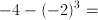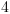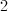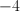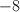Explanation:

The order of operations is PEMDAS, or (1) Parentheses (2) Exponents (3)Multiplication/Division (4) Addition/Subtraction.

First, we need to cube the quantity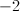.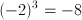Now we are left with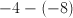.

Subtracting a negative is the same as adding a positive. So we can rewrite this as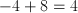### Example Question #1 : Concepts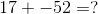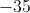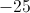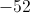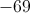Explanation:

When adding a positive to a negative number you must first pick the greater value of the two numbers while disregarding the signs.

In this case the negative number has a higher value if we don’t pay attention to the signs. Due to this fact we know our resulting number will be negative.

We then subtract the smaller number value from the higher value which gives us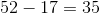Then we add the negative sign to the value to arrive at our answer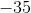### Example Question #1 : Concepts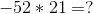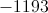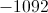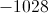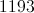Explanation:

If you multiply a negative by a positive the resulting number will be a negative number.

So we multiply the numbers together normally and then add the negative sign at the end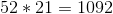The answer in this case is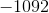### Example Question #2 : Negative Numbers

Evaluate: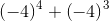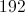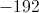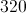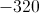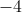Explanation:

A negative number taken to an even power is positive, and an odd number taken to an odd power is negative, so: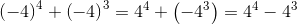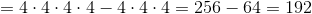### Example Question #3 : Concepts

Evaluate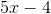for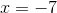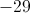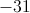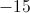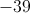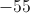Explanation: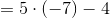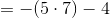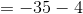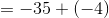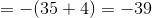### Example Question #1 : Arithmetic

Evaluate: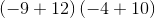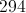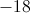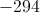Explanation: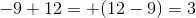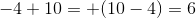Therefore,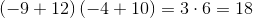### Example Question #1 : Concepts

Combine: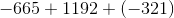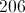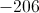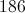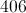Explanation:

First, combine the negative numbers. This yields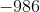.

Then, the problem is simplified to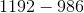. This gives you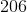### Example Question #2 : Concepts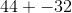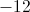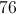Explanation:

To most effectively add a positive and negative number, ignore the signs and pick the greater of the two numbers.

In this case the positive number has a higher value if we don’t pay attention to the signs. Due to this fact we know the answer will be positive.

We then subtract the smaller number value from the higher value which gives us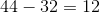Then we add the sign of the greater value to arrive at our answer.

### Example Question #2 : Arithmetic

Add: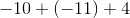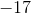Explanation:

Simply the signs before solving. A positive sign multiplied with a negative sign will convert the sign to a negative, and a negative multiplied with a negative will convert the sign to a positive.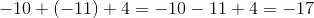### Example Question #1 : How To Multiply Negative Numbers

If a = –2 and b = –3, then evaluate a3 + b2

5

8

1

17

9

1

Explanation:

When multiplying negative numbers, we get a negative answer if there are an odd number of negative numbers being multiplied.  We get a positive answer if there are an even number of negative numbers being multiplied.

a3 + b2 becomes (–2)3 + (–3)2 which equals –8 + 9 = 1

← Previous 1 3 4 5 6 7 8 9 61 62

### All HSPT Math Resources